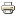# The Degree Ratio Ranking Method for Directed NetworksWorking paper
Author/s:
René van den Brink, Agnieszka Rusinowska
Issue number:
2019.09
Series:
Documents de Travail du Centre d’Economie de la Sorbonne
Publisher:
Centre d’Economie de la Sorbonne
Year:
2019
One of the most famous ranking methods for digraphs is the ranking by Copeland score. The Copeland score of a node in a digraph is the difference between its outdegree (i.e. its number of outgoing arcs) and its indegree (i.e. its number of ingoing arcs). In the ranking by Copeland score, a node is ranked higher, the higher is its Copeland score. In this paper, we deal with an alternative to rank nodes according to their out- and indegree, namely ranking the nodes according to their degree ratio, i.e. the outdegree divided by the indegree. To avoid dividing by a zero indegree, we implicitly take the out- and indegree of the reflexive digraph. We provide an axiomatization of the ranking by degree ratio using a sibling neutrality axiom, which says that the entrance of a sibling (i.e. a node that is in some sense similar to the original node) does not change the ranking among the original nodes. We also provide a new axiomatization of the ranking by Copeland score using the same axioms except that this method satisfies a different sibling neutrality. Finally, we modify the ranking by degree ratio by not considering the reflexive digraph, but by definition assume nodes with indegree zero to be ranked higher than nodes with a positive indegree. We provide an axiomatization of this ranking by modified degree ratio using yet another sibling neutrality and a maximal property. In this way, we can compare the three ranking methods by their respective sibling neutrality.
Developed by Paolo Gittoi